﻿ Bessel位势在空间L<sup>p</sup>(R<sup>n</sup>)(0 < p < 1)上的作用

# Bessel位势在空间Lp(Rn)(0 < p < 1)上的作用The Action of the Bessel Potentials on Lp(Rn)(0 < p < 1) Space

Abstract: In this paper, we concern the action of the Bessel potentials on Lp(Rn) space with (0 < p < 1). By the method of contradiction, we prove that for any r > 0, the Bessel potential cannot be a bounded linear operator from Lp(Rn) to Lr(Rn), and obtain other related results.

1. 引言

${‖uv‖}_{{L}^{r}\left(\Omega \right)}\le {‖u‖}_{{L}^{p}\left(\Omega \right)}{‖v‖}_{{L}^{q}\left(\Omega \right)},$ (1)

$p,q,r>0,\text{\hspace{0.17em}}\frac{1}{r}=\frac{1}{p}+\frac{1}{q},$ (2)

$\Omega \subset {\text{R}}^{n}$ 是一区域。值得指出的是，只要 $p,q,r$ 满足(2)式，则(1)式对任意的 $u\in {L}^{p}\left(\Omega \right)$$v\in {L}^{q}\left(\Omega \right)$ 都成立。特别地，当 $u\in {L}^{p}\left(\Omega \right)$, $v\in {L}^{q}\left(\Omega \right)$

$p,q>0,\text{\hspace{0.17em}}\frac{1}{p}+\frac{1}{q}>1$ (3)

${‖A\left(uv\right)‖}_{{L}^{r}\left(\Omega \right)}\le C\left({‖u‖}_{{L}^{p}\left(\Omega \right)},{‖v‖}_{{L}^{q}\left(\Omega \right)}\right)$ ? (P)

Bessel位势是形如

${\left(1-\Delta \right)}^{-\alpha /2},\text{\hspace{0.17em}}\alpha >0$

${K}_{\alpha }\left(x\right)={\left(4\pi \right)}^{-\frac{n}{2}}\Gamma {\left(\frac{\alpha }{2}\right)}^{-1}{\int }_{0}^{\infty }{\text{e}}^{-\frac{|x{|}^{2}}{4s}-s}{s}^{\frac{\alpha -\left(n+2\right)}{2}}\text{d}s.$ (4)

${\stackrel{^}{K}}_{\alpha }\left(\xi \right)={\left(1+|\xi |\right)}^{-\alpha /2}.$

${\left(1-\Delta \right)}^{-\alpha /2}\phi \left(x\right)={\int }_{{\text{R}}^{n}}{K}_{\alpha }\left(x-y\right)\phi \left(y\right)\text{d}y.$ (5)

${‖{\left(1-\Delta \right)}^{-\alpha /2}u‖}_{{W}^{k,p}\left({\text{R}}^{n}\right)}\le C{‖u‖}_{{L}^{1}\left({\text{R}}^{n}\right)},\text{\hspace{0.17em}}\text{\hspace{0.17em}}\forall u\in {L}^{1}\left({\text{R}}^{n}\right).$ (6)

${‖{\left(1-\Delta \right)}^{-\alpha /2}\left(uv\right)‖}_{{L}^{r}\left({\text{R}}^{n}\right)}\le {C}_{0}\left({‖u‖}_{{L}^{p}\left({\text{R}}^{n}\right)},{‖v‖}_{{L}^{q}\left({\text{R}}^{n}\right)}\right)$ (7)

${‖u‖}_{p}={\left({\int }_{{\text{R}}^{n}}{|u|}^{p}\text{d}x\right)}^{\frac{1}{p}},\text{\hspace{0.17em}}\forall u\in {L}^{p}\left({\text{R}}^{n}\right).$

${‖{\left(1-\Delta \right)}^{-\alpha /2}u‖}_{r}\le {C}_{0}\left({‖u‖}_{p}\right),\text{\hspace{0.17em}}\forall u\in {L}^{p}\left({\text{R}}^{n}\right),\text{\hspace{0.17em}}u\ge 0.$ (8)

${‖{\left(1-\Delta \right)}^{-\alpha /2}u‖}_{r}\le {C}_{0}\left({‖u‖}_{{p}_{1}},{‖u‖}_{{p}_{2}}\right),\text{\hspace{0.17em}}\forall u\in {L}^{{p}_{1}}\left({\text{R}}^{n}\right)\cap {L}^{{p}_{2}}\left({\text{R}}^{n}\right),\text{\hspace{0.17em}}u\ge 0.$ (9)

${‖{\left(1-\Delta \right)}^{-\alpha /2}\left(uv\right)‖}_{r}\le {C}_{0}\left({‖u‖}_{p},{‖v‖}_{q}\right),\text{\hspace{0.17em}}\forall u\in {L}^{p}\left({\text{R}}^{n}\right),\text{\hspace{0.17em}}v\in {L}^{q}\left({\text{R}}^{n}\right).$ (10)

${‖{\left(1-\Delta \right)}^{-\alpha /2}\left(uv\right)‖}_{r}\le {C}_{0}\left({‖u‖}_{\gamma },{‖u‖}_{p},{‖v‖}_{q}\right),\text{\hspace{0.17em}}\forall u\in {L}^{\gamma }\left({\text{R}}^{n}\right)\cap {L}^{p}\left({\text{R}}^{n}\right),\text{\hspace{0.17em}}\forall v\in {L}^{q}\left({\text{R}}^{n}\right).$ (11)

$v\in {L}^{q}\left({\text{R}}^{n}\right)$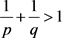时，(7)式不可能成立，从而给出了问题的否定回答。定理1.2和定理1.4则说明，

2. 定理的证明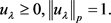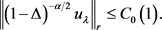(12)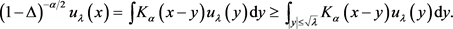(13)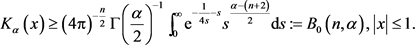(14)(15)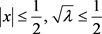(16)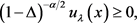(17)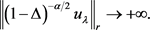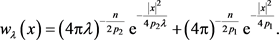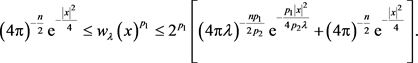(18)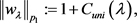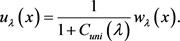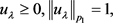(19)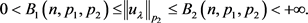(20)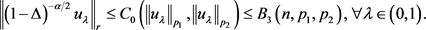(21)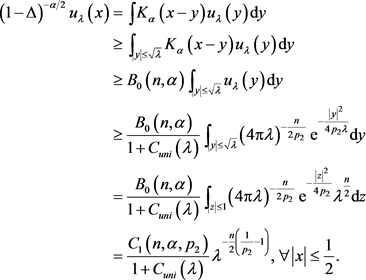(22)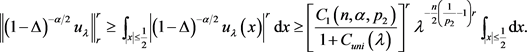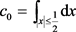，则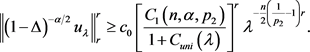(23)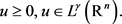(24)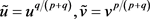，则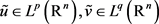。从而由(10)式知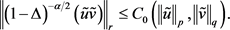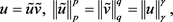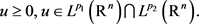(25)，则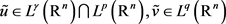。从而由(11)式知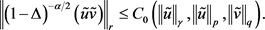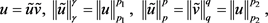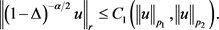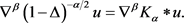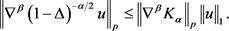(26)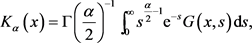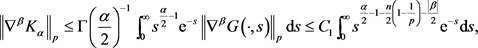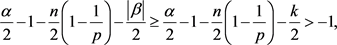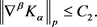(27)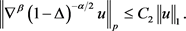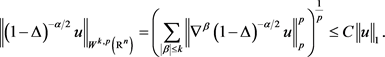Adams, R.A. and Fournier, J.J.F. (2003) Sobolev Spaces. 2nd Edition, Academic Press, New York.

 Stein, E.M. (1970) Singular Integrals and Differentiability Properties of Functions. Princeton University Press, Princeton.
https://doi.org/10.1515/9781400883882

Top